### Home > MC1 > Chapter 5 > Lesson 5.2.2 > Problem5-53

5-53.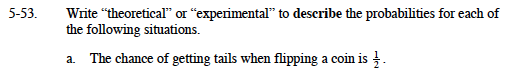If you are not sure what ''theoretical'' and ''experimental'' mean, look up the definitions.

Did someone do an experiment to make this conclusion? If not, then this probability is theoretical.

Theoretical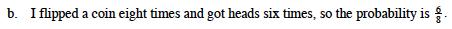Is this probability based on data from an actual test of flipping a coin?

Experimental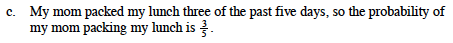Ask yourself the same questions as you did in parts (a) and (b). Remember to refer to the definitions if necessary.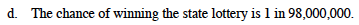Did someone actually test the probability of winning the lottery?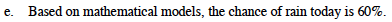One way to calculate probability without physically testing it is to use a mathematical model.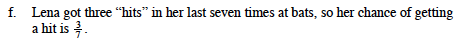How did Lena determine the probability that she would get a hit at her next at bat?